General
General
Easy

Question

# Divide the following fractions: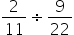##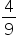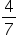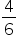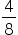Hint:

## The correct answer is:### Here, we have to divide the given fractions.Now, 2/11 ÷ 9/22= 2/11 × 22/9= 2/1 × 2/9= 2 × 2 / 1 × 9= 4/9Hence, the correct option is (a).

To remove the sign of division, we take the reciprocal of the second fraction and multiply the fraction 2/11 by the reciprocal of the second fraction.#### With Turito Foundation.#### Get an Expert Advice From Turito.